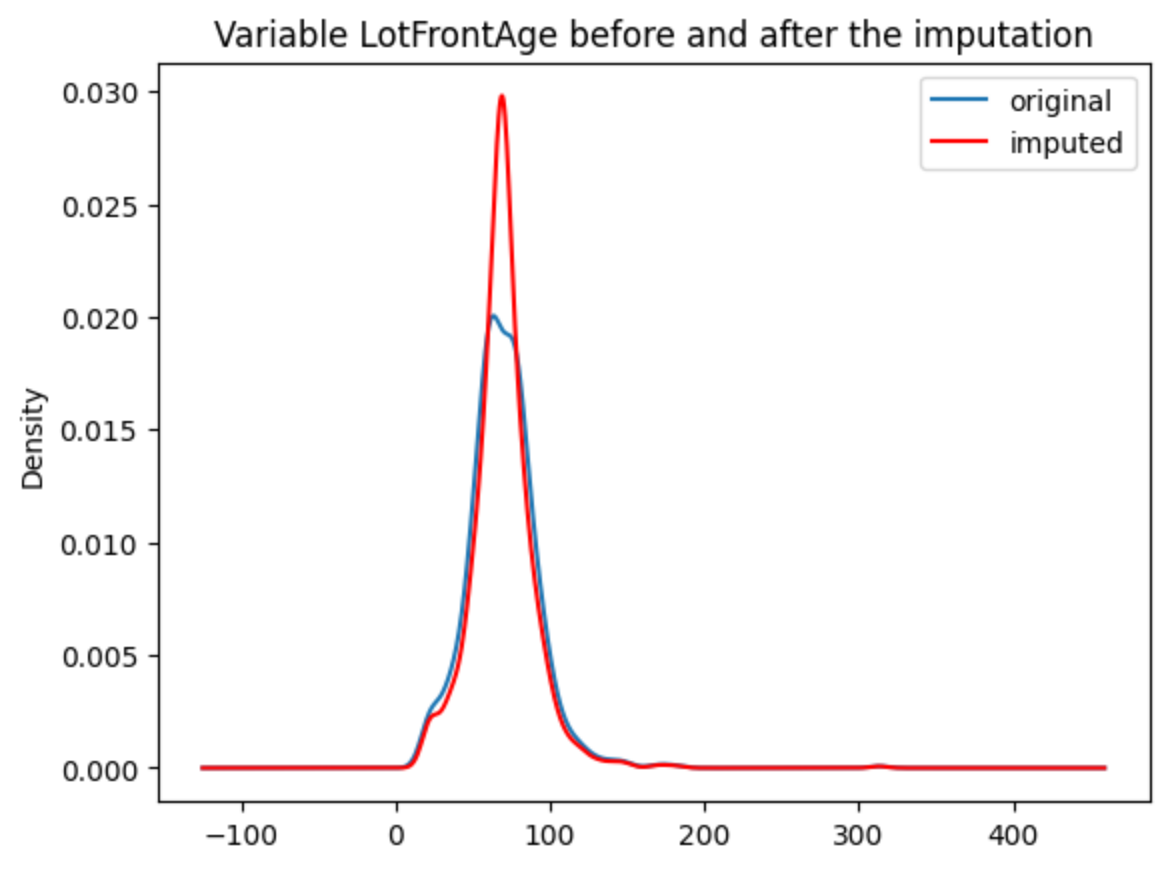# MeanMedianImputer#

The `MeanMedianImputer()` replaces missing data with the mean or median of the variable. It works only with numerical variables. You can pass the list of variables you want to impute, or alternatively, the imputer will automatically select all numerical variables in the train set.

Note that in symetrical distributions, the mean and the median are very similar. But in skewed distributions, the median is a better representation of the majority, as the mean is biased to extreme values. The following image was taken from Wikipedia. The image links to the use license.

With the `fit()` method, the transformer learns and stores the mean or median values per variable. Then it uses these values in the `transform()` method to transform the data.

Below a code example using the House Prices Dataset (more details about the dataset here).

First, let’s load the data and separate it into train and test:

```import numpy as np
import pandas as pd
import matplotlib.pyplot as plt
from sklearn.model_selection import train_test_split

from feature_engine.imputation import MeanMedianImputer

# Separate into train and test sets
X_train, X_test, y_train, y_test = train_test_split(
data.drop(['Id', 'SalePrice'], axis=1),
data['SalePrice'],
test_size=0.3,
random_state=0,
)
```

Now we set up the `MeanMedianImputer()` to impute in this case with the median and only 2 variables from the dataset.

```# set up the imputer
median_imputer = MeanMedianImputer(
imputation_method='median',
variables=['LotFrontage', 'MasVnrArea']
)

# fit the imputer
median_imputer.fit(X_train)
```

With fit, the `MeanMedianImputer()` learned the median values for the indicated variables and stored it in one of its attributes. We can now go ahead and impute both the train and the test sets.

```# transform the data
train_t= median_imputer.transform(X_train)
test_t= median_imputer.transform(X_test)
```

Note that after the imputation, if the percentage of missing values is relatively big, the variable distribution will differ from the original one (in red the imputed variable):

```fig = plt.figure()In the following Jupyter notebook you will find more details on the functionality of the `MeanMedianImputer()`, including how to select numerical variables automatically. You will also see how to navigate the different attributes of the transformer to find the mean or median values of the variables.